## Working with Logic Analyzer in PSLab Android app

This blog demonstrates the working of Logic Analyzer instrument available in PSLab Android app. It also includes a detailed description of the features available in the Logic Analyzer instrument along with a step by step guide on how to work with it which will be beneficial to first-time users of the PSLab application.

The functionality of the Logic Analyzer available in PSLab Android app is same as that in PSLab Desktop App. So, it would be easy for a user of PSLab Desktop Application to get acquainted with this Logic Analyzer. The only difference in this instrument is the changed and attractive UI which makes working with it very easy.

## Why use Logic Analyzer?

The Logic Analyzer instrument provides the functionality of capturing and plotting the digital waves on the screen so that it would be easy for a user to determine the time relationship between different waves. So, this instrument would be very useful while working with timing diagrams, protocol decodes, state machines traces, assembly language, or with source-level software.

## How to generate different digital pulses in the PSLab app?

Logic Analyzer needs to be provided with some input of digital pulses among whom time relationship is to be found out. Digital pulses generated from different systems can be directly provided as input to the Logic Analyzer for analyzing. But PSLab provides a functionality to generate digital pulses up to some constrained frequency.

Following are the steps to generate different digital waves in PSLab Android application :

• Open PSLab Android application and click on the Wave Generator tile as shown in figure 1. After opening the instrument, the screen will look as shown in figure 2.Figure 1. Wave Generator instrument tile available in PSLab Android appFigure 2. The main screen of the Wave Generator instrument

• Click on the MODE button to change the mode to PWM. The screen will look as shown in figure 3.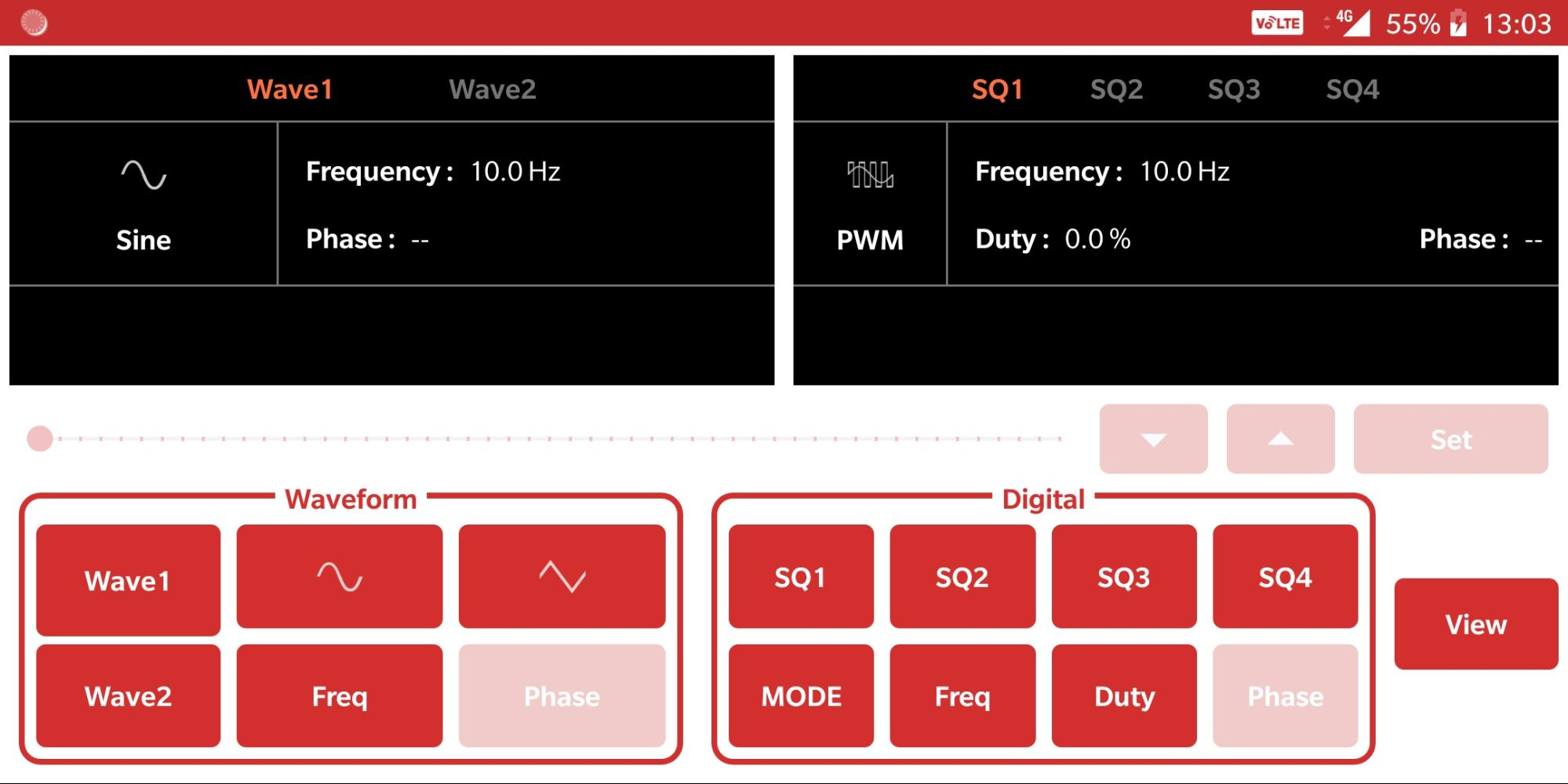Figure 3. PWM mode in Wave Generator

• PSLab device provides generation of maximum four digital waves at once. In this example, I will proceed by utilizing only two pins i.e. SQR1and SQR2 (where SQR = Acronym of square wave generator and the number next to it is the pin ID available on the PSLab device) to demonstrate the working of Two Channel Mode in Logic Analyzer. Set the duty cycles and frequency for the selected pins as desired (try to keep all the duty cycles different from each other to understand the process of measurement easily).

NOTE: User can also set phase angle for different waves but I will proceed with defaults.

## How to analyze the generated waves in Logic Analyzer?

• Now go back and select the Logic Analyzer tile as shown in figure 4 from the list of available instruments. A screen as shown in figure 5 should open.Figure 4. Tile of Logic Analyzer instrument available in the PSLab app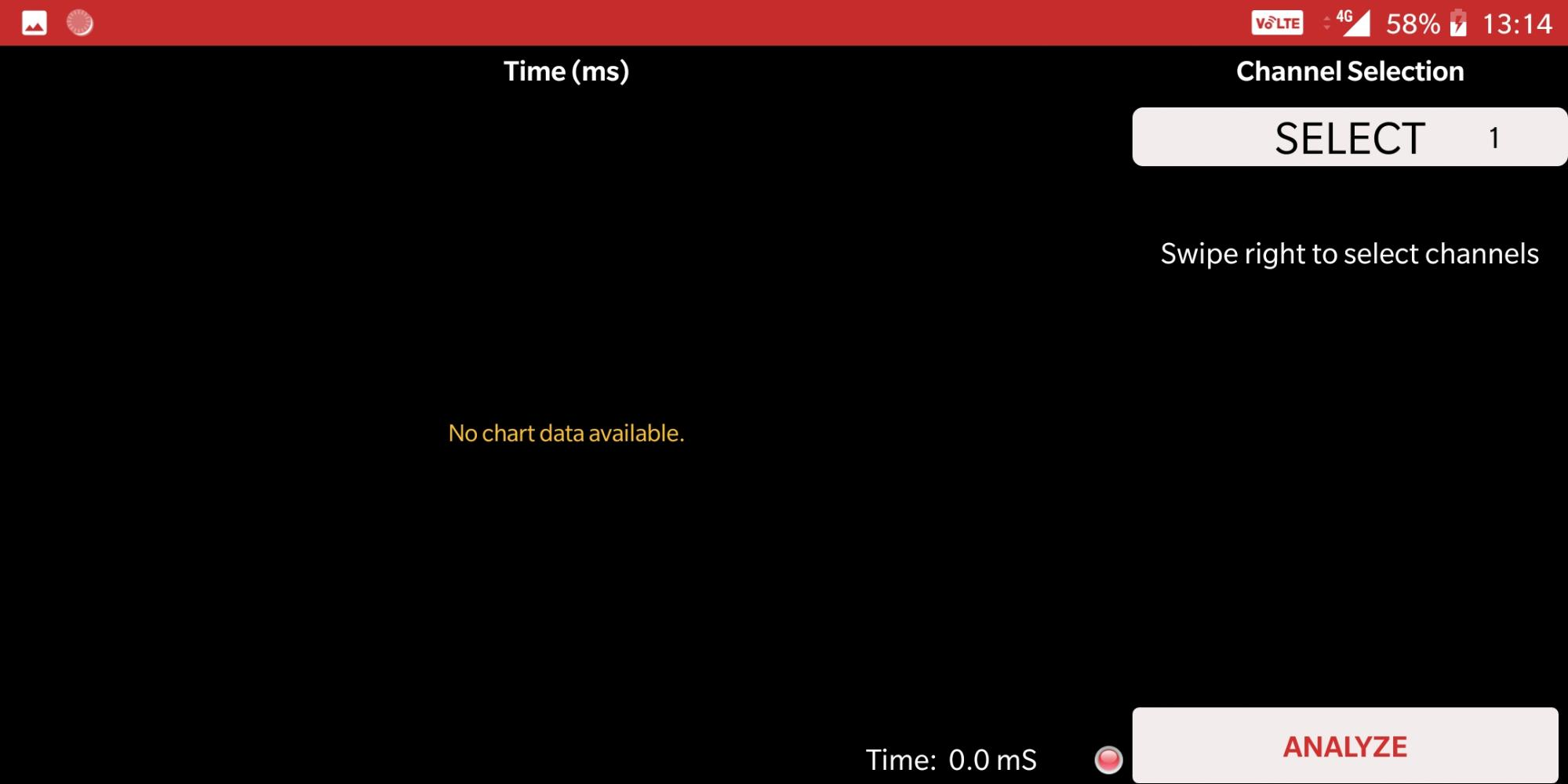Figure 5. The main screen of the Logic Analyzer instrument

On the right-hand side, you can see a slider whose initial value is SELECT which shows the information on how to use the slider below it. Below the Channel Selection area is the Analyze button used to fetch and plot the data which is generated or provided to the respective Logic Analyzer pins i.e. ID1, ID2, ID3, and ID4.

The blank area on the left is where the graph will be plotted after fetching data points. Below it is the Axis Indicator used to indicate the position of the highlighter so that time measurement can be done easily. To the right of the Axis Indicator is a small light which indicates the status of the device. It turns GREEN if the device is connected else it remains in RED.

• In this blog, I will demonstrate the Two Channel Mode. But all the other available modes need the same implementation by only varying the number of pins in use. So, slide to 2 in the Carousel View and a screen as shown in figure 6 will pop up.Figure 6. Two Channel Mode in Logic Analyzer

• Connect the wires on the PSLab device as shown in Figure 7.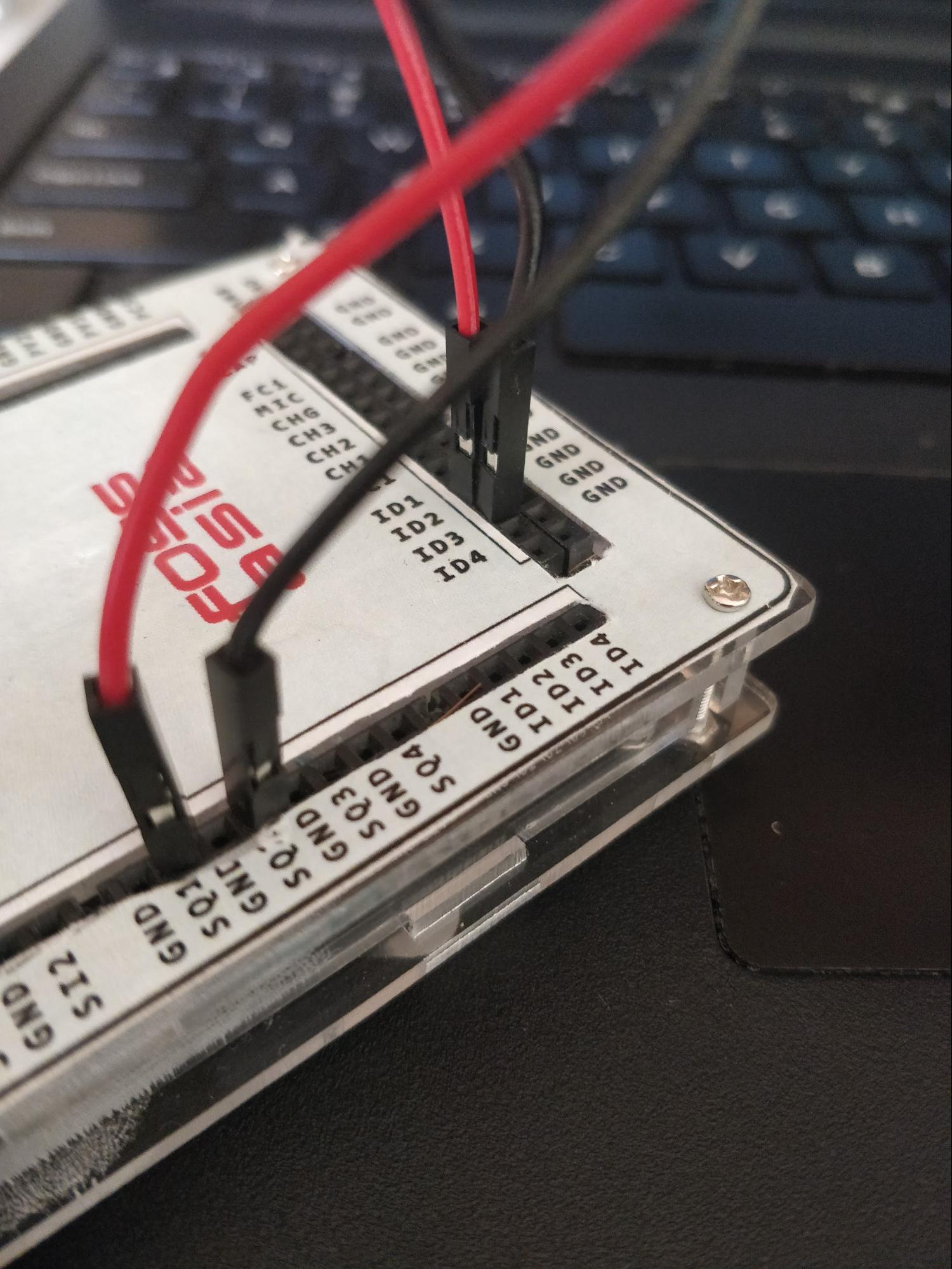Figure 7. Connecting wires on PSLab

NOTE: The Logic Analyzer pins used in this demonstration are ID1 and ID2. But any IDx pin can be chosen for analysis. But try to maintain the selected choice throughout the implementation.

• Now from the Channel Selection area, select the channel for the first card to  ID1 (default) and that for the second card to ID2. For Edges Selection, maintain the defaults.

NOTE: There are several options available for plotting the digital waves besides the default selected i.e.

1. Every Edge – Plot every edge of the signal
2. Falling Edges – Plot only falling edges of the signal (When a signal comes from 1 to 0 state)
3. Rising Edges Only – Plot only rising edges of the signal (When a signal comes from 0 to 1 state)
4. Disabled – Don’t plot the selected wave
• After Channel Selection, press the Analyze button to plot the data. On pressing the Analyze button, a circular loading sign will appear showing that the data is being fetched and converted to data that can be plotted. As soon as the data is ready to be plotted, the loading sign vanishes and the graph appears as shown in figure 8.• The time relationship between the plotted data can be found out by clicking over the rising/falling edges and noting the time shown in the Axis Indicator as shown in figure 8.
• An example of Edge Selection is shown in figure 9.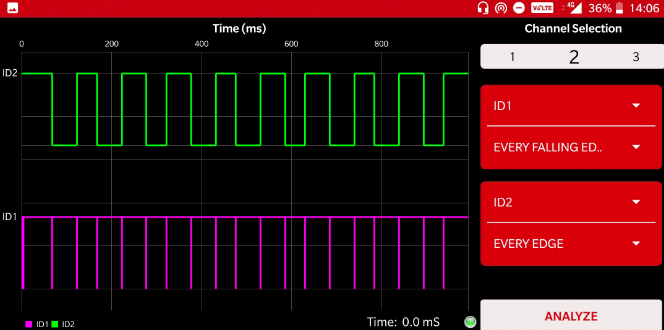Figure 9. Example of Edge Selection option

Here, EVERY FALLING EDGE option is selected for the ID1 channel and EVERY RISING EDGE option is selected for the ID2 channel.

So in this way, the Logic Analyzer instrument available in PSLab Android application can be used to ease out the process of calculating the time interval between different edges for different/same digital pulse/s.

## Resources

1. PSLab Android Application – https://github.com/fossasia/pslab-android (Link to repo)
2. PSLab device pins sticker – https://github.com/fossasia/pslab-artwork/blob/master/Sticker/pslabdesign.png

## Working with Logic Analyzer in PSLab application

This blog demonstrates the working of Logic Analyzer instrument available in PSLab Desktop Application. It also includes a detailed description of the features available in the Logic Analyzer instrument. Also, it provides a step by step guide on how to work with the Logic Analyzer provided by PSLab which will be beneficial to first-time users.

## What is a Logic Analyzer?

A Logic Analyzer is an electronic instrument used to capture and display digital signals with an added functionality of providing the time difference between different edges of different pulses. It is mainly used to observe the time relationship between different digital signals. An example of a standard Logic Analyzer available in the market is as shown in figure 1.Figure 1. Standard Logic Analyzer

## How to generate different digital pulses in PSLab?

Logic Analyzer needs to be provided with some input of digital pulses among whom time relationship is to be found out. Digital pulses generated from different systems can be directly provided as input to the logic analyzer for analyzing. But PSLab provides a functionality to generate digital pulses up to some constrained frequency.

Following are the steps to generate different digital waves in the PSLab desktop application :

• Go to Advanced Control Section of PSLab app. The screen should look like one as shown in Figure 2.• PSLab device provides generation of maximum four digital waves at once. In this example, I will proceed by utilizing all the four pins i.e. SQR1, SQR2, SQR3, SQR4 (where SQR = Acronym of square wave generator and the number next to it is the pin ID available on the PSLab device). Set the duty cycles for each of the pins as desired (try to keep all the duty cycles different from each other to understand the process of measurement easily). After setting it should look something like Figure 3.Figure 3. Configuring PWM

NOTE: User can also set phase angle for different waves but I will proceed keeping all without any phase difference.

• Now set the frequency of the digital waves in the tab provided next to text Frequency and then press the SET button. This should generate desired digital waves when connected.

## How to analyze the generated waves in Logic Analyzer?

• Now go to the Experiments section and click on the Logic Analyzer instrument as shown in Figure 4.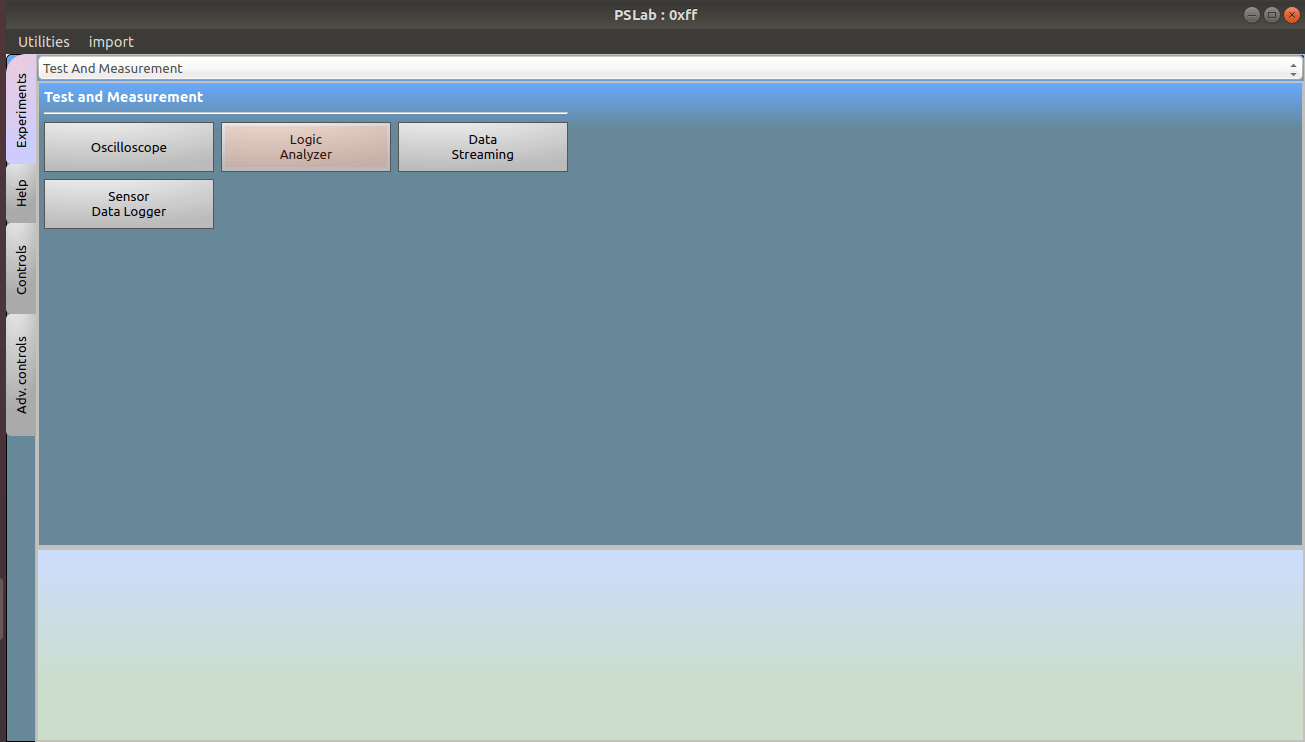Figure 4. Test and Measurement Page

• Now a screen as shown in Figure 5 should open which is the main screen for Logic Analyzer Instrument.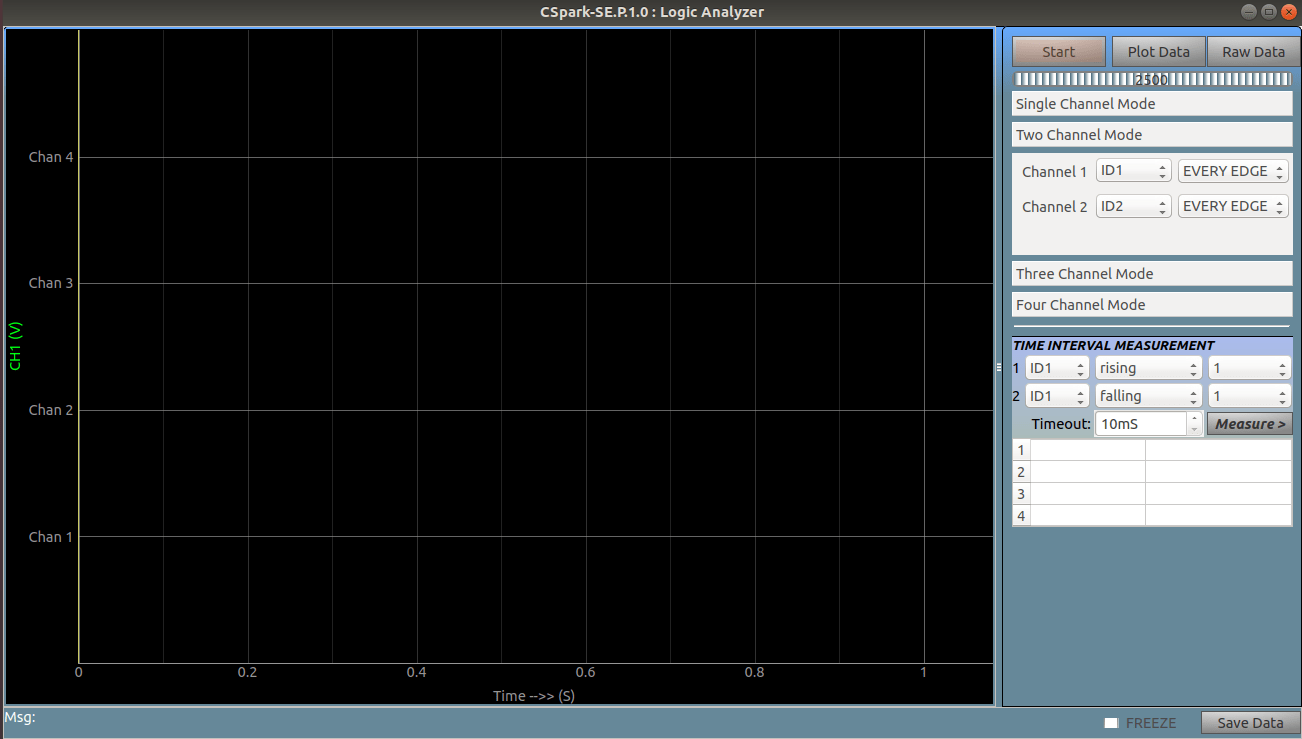Figure 5. Logic Analyzer Main Page

On the right, you can see three buttons i.e Start, Plot Data and Raw Data. Below that selection for the number of channels is provided. And at last, the time measurement tool is provided which can measure the time difference between different edges of different digital waves. The graph at the center is the place where all the waves generated will be plotted.

• Now as we have generated four different waves, we need to navigate to Four Channel Mode in the Channel Selection section. There you can observe four pins i.e. ID1, ID2, ID3, and ID4 are selected by default as shown in Figure 6. So, we need to connect the pins SQR1, SQR2, SQR3 and SQR4 with pins ID1, ID2, ID3 and ID4 on the PSLab device.Figure 6. Channel Selection Section

NOTE: There are several options available for plotting the digital waves besides the one selected in the above image i.e.

1. Every Edge – Plot every edge of the signal
2. Falling Edges – Plot only falling edges of the signal (When a signal comes from 1 to 0 state)
3. Rising Edges Only – Plot only rising edges of the signal (When a signal comes from 0 to 1 state)
4. Disabled – Don’t plot the selected waveFigure 7. Connecting wires on PSLab device

• Connect the wires on the PSLab device as shown in Figure 7.
• Now, as the device is connected, we can use the three buttons located at the top right corner to plot the digital waves. The functionalities of all the three buttons are as follows:
1. Start – It collects exactly 2500 sample points from the wave generated to be plotted
2. Plot Data – It plots the data on the graph if the samples are collected successfully
3. Raw Data – It provides a sheet containing time at particular intervals and the difference between the data points at that particular time
• Press the Start button and as soon as the samples are collected, press the Plot Data button. Then the screen should look something like Figure 8.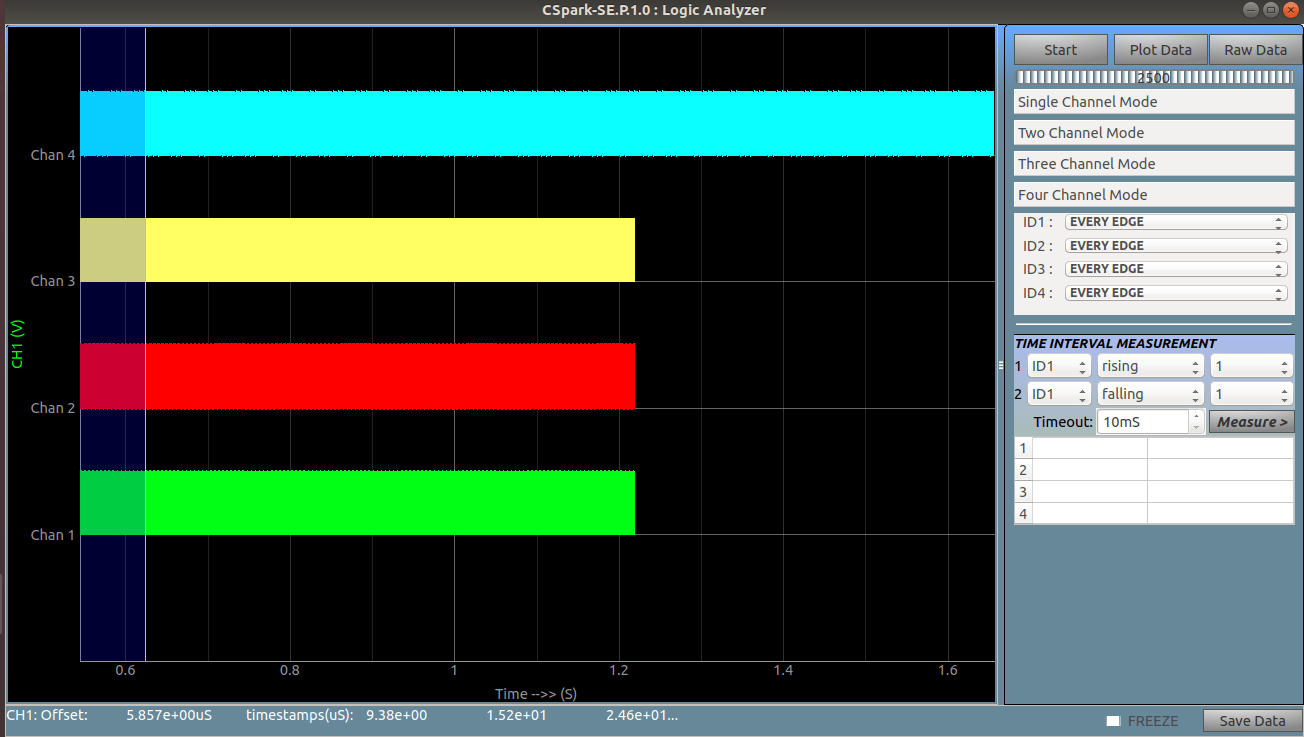Figure 8. Final graph of input provided

Zoom in further, and the screen will look like Figure 9.Figure 9. Zoomed In graph for better visualization

• So now, the waves are plotted and are ready to be analyzed. Time difference between any two edges for any two distinct or a similar wave can be found out by using the TIME INTERVAL MEASUREMENT TOOL which is as shown in Figure 10.Figure 10. Time Interval Measurement Tool

• The working of this tool can be explained by taking an example as follow :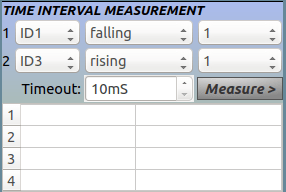Figure 11. Configuring the Time Interval Measurement Tool

Suppose time difference between the rising edge of ID1 and falling edge of ID3 is to be measured. So, set the parameters as shown in Figure 11 for the following situation.

The timeout of the Logic Analyzer can be set to any desired value from 10mS to 9999 mS. Here, I will proceed with 10mS of the timeout. Now, press the measure button to see the result. It will be same as the Figure 12 shown below if all the configurations are set as the one in the  example :Figure 12. Calculated time difference displayed

So in this way, many calculations can be taken out very easily and can be viewed at once at a single place without manually doing any calculations. Also, the data generated can be stored in the local system by pressing the Save Data button located at the bottom right corner.

## Resources

1. PSLab Desktop Application – https://github.com/fossasia/pslab-desktop-apps (Link to repo)
2. PSLab device pins sticker – https://github.com/fossasia/pslab-artwork/blob/master/Sticker/pslabdesign.png

## Plotting Digital Logic Lines In PSLab Android App

The PSLab device offers the Logic Analyzer functionality. A Logic Analyzer is a laboratory instrument that can capture and display digital signals from a digital system or circuit. It is similar to what an oscilloscope is for analog signals and is used to study timing relationship between different logic lines. It plots the logic lines/timing diagram which tells us the information about the state of the Digital System at any instant of time. For example, in the image below we can study the states of digital signals from channels ID1, ID2, ID3 at different times and find parameters like the propagation delay. It’s also used to find errors in Integrated Circuits (ICs) and debug logic circuits.How I plotted ideal logic lines using MPAndroid Chart library?

Conventional method of adding data points results in the plot as illustrated in the image below. By conventional method I mean basically adding Y-axis (logic state) values corresponding to X-axis values (timestamp).

In the above plot, logic lines follow non-ideal behaviour i.e they take some time in changing their state from high to low. This non-ideal behaviour of these lines increases when the user zooms in graph to analyse timestamps.

Solution to how we can achieve ideal behaviour of logic lines:

A better solution is to make use of timestamps for generating logic lines i.e time instants at which logic made a transition from HIGH -> LOW or LOW -> HIGH. Lets try to figure out with an example:

Timestamps = { 1, 3, 5, 8, 12 } and initial state is HIGH ( i.e at t = 0, it’s HIGH ). This implies that at t = 1, transition from HIGH to LOW took place so at t = 0, it’s HIGH, t = 1 it’s both HIGH and LOW,  at t = 2 it’s LOW.
Now at t = 0 & t = 2, you can simple put y = 1 and 0 respectively. But how do you add data-point for t = 1. Trick is to see how transition is taking place, if it’s HIGH to LOW then add first 1 for t = 1 and then 0 for t = 1.
So the set of points look something like this:

( Y, X ) ( LOGIC , TIME ) -> ( 1, 0 ) ( 1, 1 ) ( 0, 1) ( 0, 2 ) ( 0, 3 ) ( 1, 3 )  ( 1, 4 ) …

Code snippet for adding coordinates in this fashion:

```int[] time = timeStamps.get(j);
for (int i = 0; i < time.length; i++) {
if (initialState) {
// Transition from HIGH -> LOW
} else {
// Transition from LOW -> HIGH
}

// changing state variable
initialState = !initialState;
}```

After adding data-points in above mentioned way, we obtained ideal logic lines successfully as illustrated in the image given below## Environment Monitoring with PSLab

In this post, we shall explore the working principle and output signals of particulate matter sensors, and explore how the PSLab can be used as a data acquisition device for these.

### Working Principle

A commonly used technique employed by particulate matter sensors is to study the diffraction of light by dust particles, and estimate the concentration based on a parameter termed the ‘occupancy factor’. The following image illustrates how the most elementary particle sensors work using a photogate, and a small heating element to ensure continuous air flow by convection.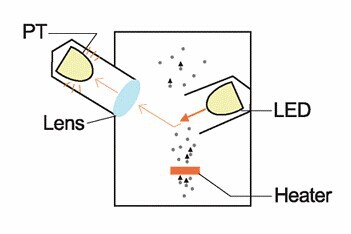### Occupancy Rate

Each time a dust particle of aerodynamic diameters 2.5um passes through the lit area, a phenomenon called Mie scattering which defines scattering of an electromagnetic plane wave by a homogenous sphere of diameter comparable to the wavelength of incident light, results in a photo-signal to be detected by the photosensor.  In more accurate dust sensors, a single wavelength source with a high quality factor such as a laser is used instead of LEDs which typically have broader spectra.

The signal output from the photosensor is in the form of intermittent digital pulses whenever a particle is detected. The occupancy ratio can be determined by measuring the sum total of time when a positive signal was output from the sensor to the total averaging time. The readings can be taken over a fairly long amount of time such as 30 seconds in order to get a more accurate representation of the occupancy ratio.

### Using the Logic analyzer to capture and interpret signals

The PSLab has a built-in logic analyzer that can acquire data signals up to 67 seconds long at its highest sampling rate, and this period is more than sufficient to record and interpret a dataset from a dust sensor. An inexpensive dust sensor, DSM501A was chosen for the readings, and the following results were obtained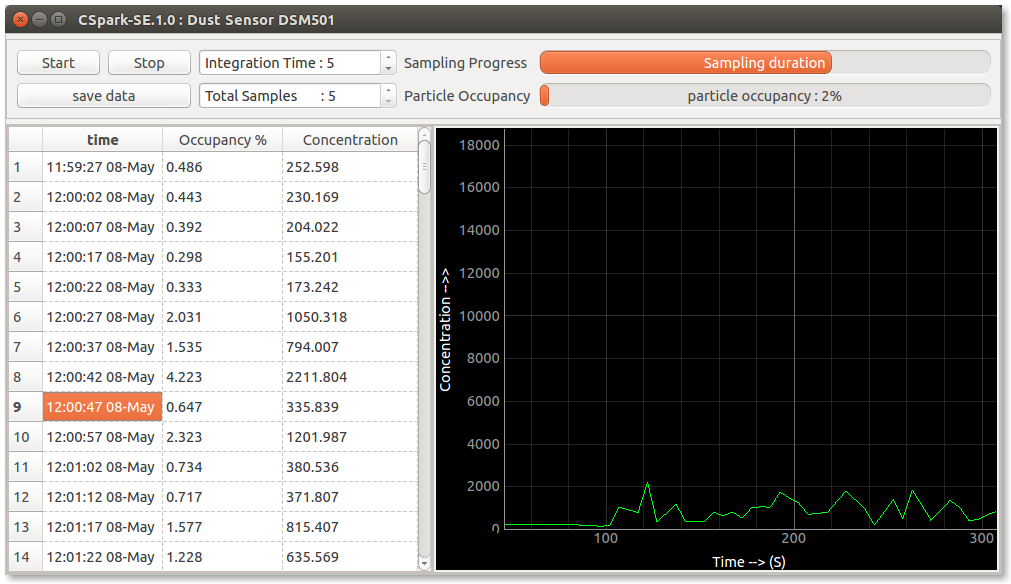Dust sensor readings from an indoor, climate controlled environment. After the 100 second mark, the windows were opened to expose the sensor to the outdoor environment.

A short averaging time has resulted in large fluctuations in the readings, and therefore it is important to maintain longer averaging times for stable measurements.

### Recording data with a python script instead of the app

The output of the dust sensor must be connected to ID1 of the PSLab, and both devices must share a common ground which is a prerequisite for exchange of DC signals. All that is required is to start the logic analyzer in single channel mode, wait for a specified averging time, and interpret the acquired data

Record_dust_sensor.py

```from PSL import sciencelab   #import the required library
import time
import numpy as np
I = sciencelab.connect()           #Create the instance
I.start_one_channel_LA(channel='ID1',channel_mode=1,trigger_mode=0)  #record all level changes
time.sleep(30)   #Wait for 30 seconds while the PSLab gathers data from the dust sensor
a,_,_,_,e =I.get_LA_initial_states()      #read the status of the logic analyzer
raw_data =I.fetch_long_data_from_LA(a,1)  #fetch number of samples available in chan #1
stamps =I.dchans.timestamps    #Obtain a copy of the timestamps
if len(stamps)>2:   #If more than two timestamps are available (At least one dust particle was detected
if not self.I.dchans.initial_state:   #Ensure the starting position of timestamps
stamps = stamps[1:] - stamps   # is in the LOW state
diff = np.diff(stamps)   #create an array of individual time gaps between successive level changes

lows = diff[::2]      #Array of time durations when a particle was not present
highs = diff[1::2]    #Array of time durations when a particle was present
low_occupancy = 100*sum(lows)/stamps[-1] #Occupancy ratio
print (low_occupancy) # datasheets of individual dust sensors also provide a mathematical
#equation to interpret the occupancy ratio as concentration of
#particulate matter
```